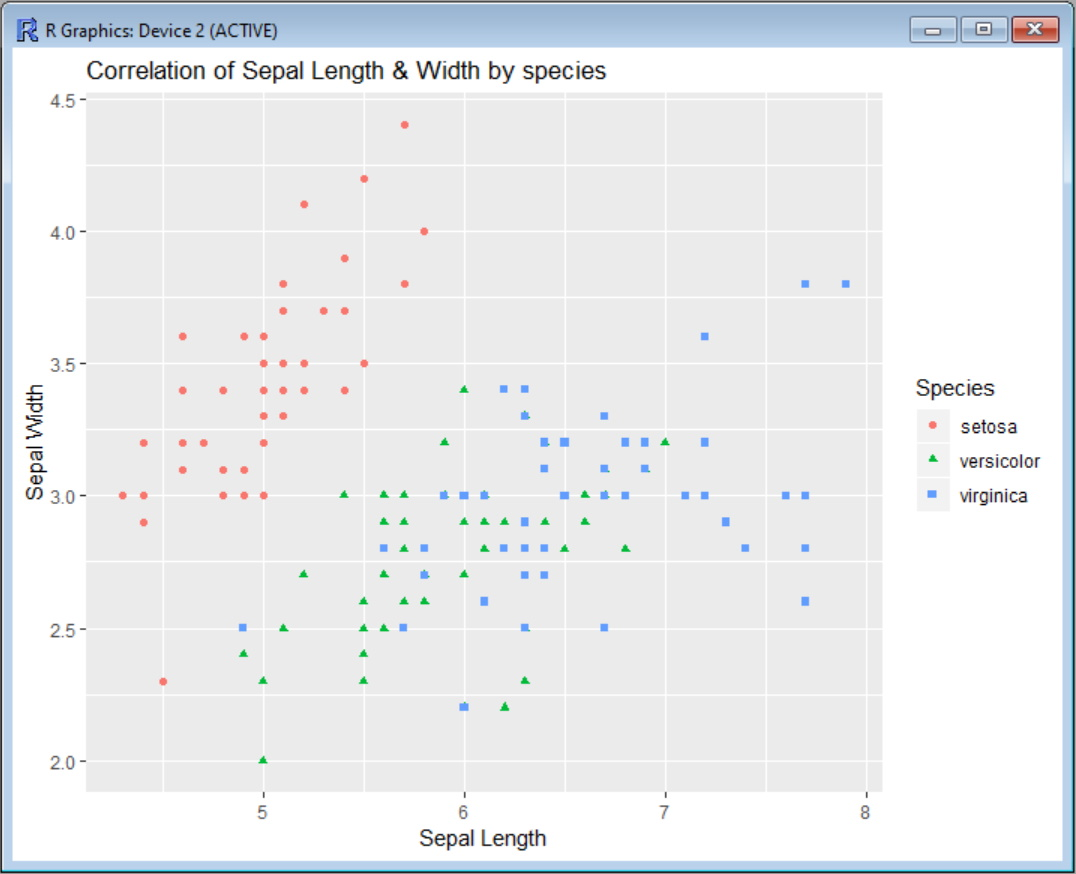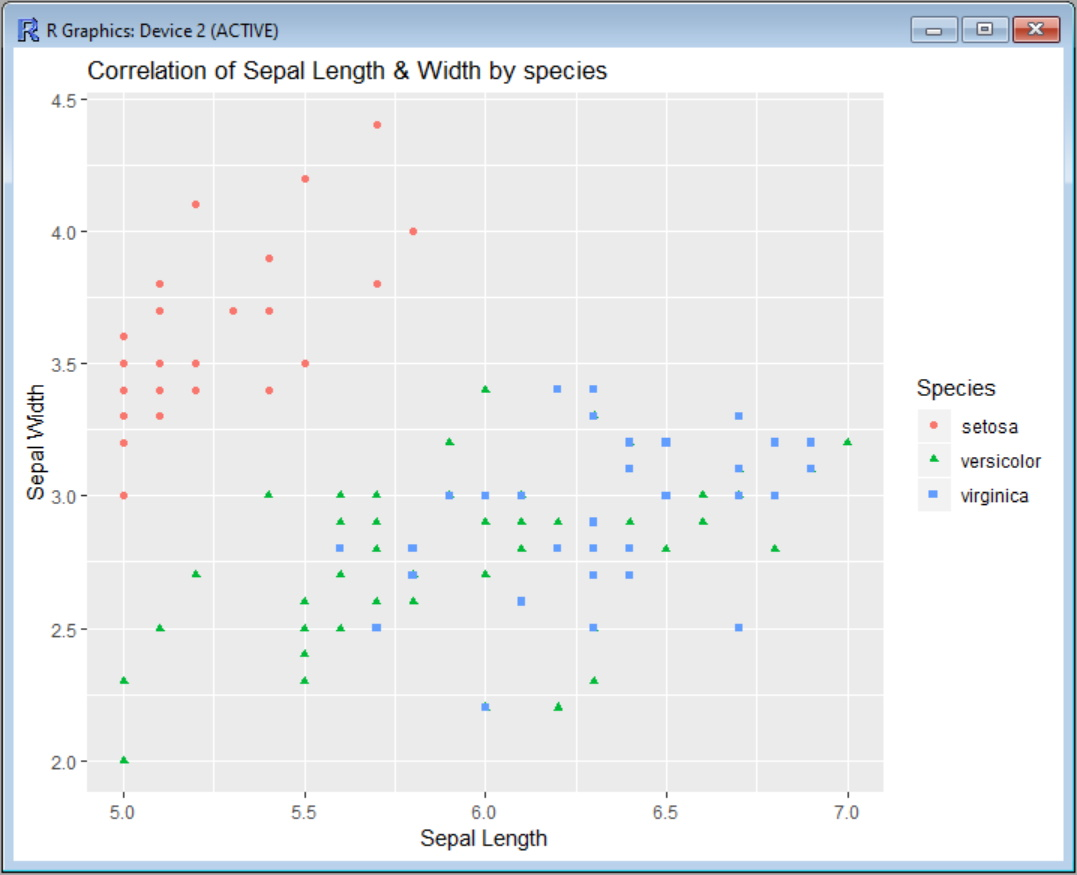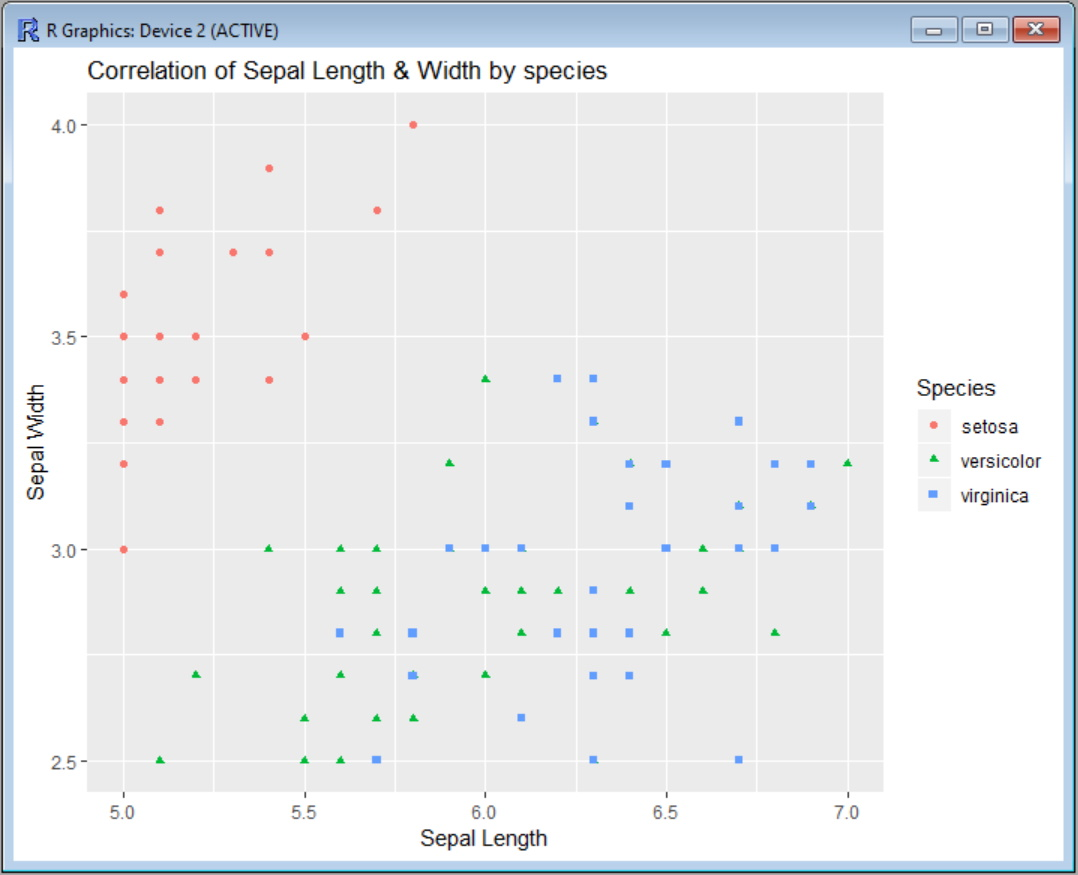# Set Axis Limits in Ggplot2 R Plots

Gustavo du Mortier Mar 29, 2022 Dec 19, 2020

Let’s first see our scatter plot without putting any limit to the axis. In this example, we use the Iris data set to correlate the sepal width values and sepal width, categorizing the species by color. Before calling the `ggplot` function, we need to install the `ggplot2` package and load the corresponding library:

``````install.packages("ggplot2")
library(ggplot2)
scatter <- ggplot(data=iris, aes(x = Sepal.Length, y = Sepal.Width))
scatter + geom_point(aes(color=Species, shape=Species)) +
ylab("Sepal Width") + xlab("Sepal Length") +
ggtitle("Correlation of Sepal Length & Width by species")
``````

Output:Now, suppose we want to limit the x-axis (Sepal Length) so that the plot shows only the region between `x=5` and `x=7`. We have two options to do that: using `scale_x_continuous` or using `coord_cartesian`.

`scale_x_continuous` removes all data points that fall outside the specified range for the given axis, while `coord_cartesian` only adjusts the visible area. In most cases, both options will render the same results. But if you happen to fit something to the data, the fitted values would probably change.

## Set X-Axis Limits With `scale_x_continuous` in `ggplot2`

To use `scale_x_continuous`, you need to specify a vector with the lower and upper limits of the x-axis, like this:

``````scatter <- ggplot(data=iris, aes(x = Sepal.Length, y = Sepal.Width))
scatter + geom_point(aes(color=Species, shape=Species)) +
ylab("Sepal Width") + xlab("Sepal Length") +
ggtitle("Correlation of Sepal Length & Width by species") +
scale_x_continuous(limits = c(5, 7))
``````

Output:You will also get a warning message telling you how many data points are eliminated from the graph:

``````Warning message:
Removed 34 rows containing missing values (geom_point).
``````

In case you also want to limit the y-axis, you can add `scale_y_continuous` the same way, as in this example:

``````![Scatter-plot-with-limits-both-axis](C:\GDM\Cosas\UpWork\Dishan Consultancy\R\Scatter-plot-with-limits-both-axis.jpg)scatter <- ggplot(data=iris, aes(x = Sepal.Length, y = Sepal.Width))
scatter + geom_point(aes(color=Species, shape=Species)) +
ylab("Sepal Width") + xlab("Sepal Length") +
ggtitle("Correlation of Sepal Length & Width by species") +
scale_x_continuous(limits = c(5, 7)) +
scale_y_continuous(limits = c(2.5, 4))
``````

The output will show the limits on both axis:You can also use the shorthand functions `xlim` and `ylim` instead of `scale_x_continuous` and `scale_y_continuous` to make the command a little shorter:

``````scatter <- ggplot(data=iris, aes(x = Sepal.Length, y = Sepal.Width))
scatter + geom_point(aes(color=Species, shape=Species)) +
ylab("Sepal Width") + xlab("Sepal Length") +
ggtitle("Correlation of Sepal Length & Width by species") +
xlim(5, 7) + ylim(2.5, 4)
``````

## Use `coord_cartesian` to Limit Both Axes in `ggplot2`

If you want to use `coord_cartesian` instead of `scale_x_continuous` and `scale_y_continuous`, the command would be like this:

``````scatter <- ggplot(data=iris, aes(x = Sepal.Length, y = Sepal.Width))
scatter + geom_point(aes(color=Species, shape=Species)) +
ylab("Sepal Width") + xlab("Sepal Length") +
ggtitle("Correlation of Sepal Length & Width by species") +
coord_cartesian(xlim = c(5, 7), ylim = c(2.5, 4))
``````

And the plot output will be the same.

In the RStudio cheatsheet of ggplot2, you can find visual clarification for the use of all the options for this command.